Next: Inference Up: Class Distributions Previous: Class Distributions

## Classifying SPMs in FMRI

The mixture models could be used as a second stage to analyze the SPMs resulting from a first stage of a univariate temporal FMRI analysis (Woolrich et al., 2001). This is the same two-stage approach used when using the commonly used random field theory of Worsley et al. (1992) and is also the same approach that Everitt and Bullmore (1999); Hartvig (2000) take with the mixture models they consider.

The result of a univariate temporal analysis is effectively the parameter estimate,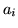, of the correlation with the assumed response at each voxel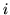. We might consider usingas the observations,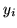, for our mixture models. However, this carries over none of the uncertainty in the estimation offrom the univariate temporal analysis. Hence, we use instead the normalised version: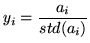(20)

See Woolrich et al. (2001) for one way of estimatingand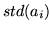using a univariate temporal analysis.

We now need to specify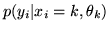for all classes. For this we propose to use a similar approach to Hartvig and Jensen (2000), who uses three classes: activation, deactivation and non-activation. The non-activating class is modelled as a Normal distribution:(21)

where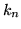represents the label for the non-activating class. To reflect the assumption that the activating class can only have positive values of, we use a Gamma function for the activation component: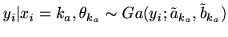(22)

where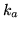represents the label for the activating class. For the deactivating class, we also use a Gamma function: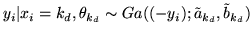(23)

where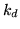represents the label for the deactivating class.

Note that we sometimes find it useful for the interpretation of parameters to reparameterise a Gamma distribution in terms of its mean,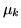, and variance,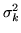. See the appendix for this transformation.

The hyperpriors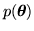to be used on the component parameters are non-informative, disperse priors. However, we do place a restriction on the mode of the activation and deactivation Gamma classes. The mode of the activation class is constrained to be greater than the mean of the non-activation class, and the mode of the deactivation class is constrained to be less than the mean of the non-activation class. This encodes a sensible prior belief about the expected shape of the activation and deactivation distributions with respect to the non-activation. The mode of a Gamma distribution is given in equation 27.Next: Inference Up: Class Distributions Previous: Class Distributions